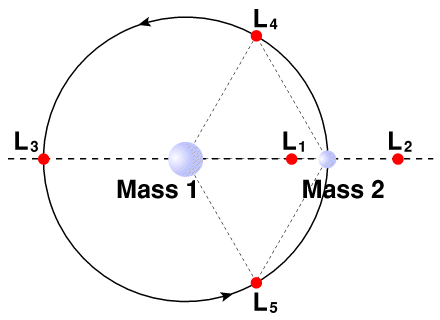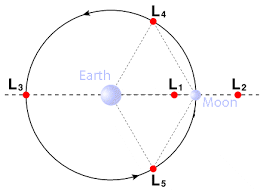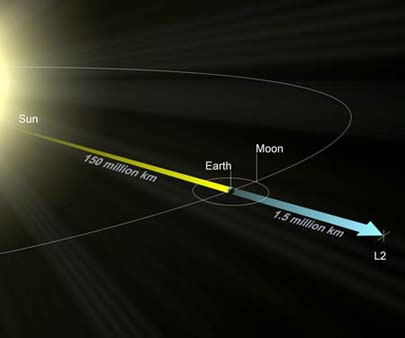# Lagrange Points

What is a Lagrange point? While reading Robot Dawn: The Mazzy Nova Chronicles, you will come across the term many times, and the characters will even visit a couple. Lagrange points are located within all two-body gravitational systems, where a smaller body orbits a much larger one in a circular orbit. Lagrange points are positions in space where the centrifugal force on the orbiting body and the converging gravities from the two heavenly bodies oppose each other such that they create special gravitational conditions for anything located there. See the figure below. Mass 2 is in orbit (the big circle) about Mass 1. This results in the gravitational forces at Lagrange points being configured such that a satellite positioned there orbits the larger mass in formation with the smaller one. Lagrange points never change position relative to each other or the two masses. They are sometimes termed “parking lots” in space.

Lagrange Point LocationsGeneral positions of Lagrange points that maintain orientation relative to Mass 2 as it orbits  (counterclockwise) about Mass 1.

Five Lagrange points exist within any two-body gravitational system. The notation for these points is developed using the first letter of each body, the large body’s first, as follows:

Sun-Earth Lagrange points: SE-L1, SE-L2, SE-L3, SE-L4, and SE-L5
Sun-Mars Lagrange points: SM-L1-5
Sun-Jupiter Lagrange points: SJ-L1-5
Earth-Moon Lagrange points: EM-L1-5

And so on.

Lagrange Points 1, 2, and 3

The first three Lagrange points lie along a line connecting the centers of the two-body system. L1, as it is called, is between the two bodies but closer to the smaller body. L2 is out beyond the smaller orbiting body, and L3 is behind the larger body, opposite the smaller body and is also positioned on the orbit of Mass 2.

Another property of these three Lagrange points is that they are unstable. In other words, if a satellite is put at one of these Lagrange points, it will drift off of the Lagrange point and tend to want to go even farther away. Because of the instability, a satellite positioned there will require considerable energy to maintain its position, quite a lot of station-keeping.

Lagrange Points 4 and 5

The other two Lagrange points do not lie along the connecting line but are still within the orbital plain. They are off the line but do lie on, or in the vicinity of, the orbit of the smaller body. If we draw a line from the center of the larger object at an angle of 60 degrees above the line, then where it intersects the smaller body’s orbit is where L4 is located. L5 is located at 60 degrees below the line, again on the smaller body’s orbit. The locations of these five Lagrange points then orbit with the smaller body and maintain their positions relative to it throughout its orbit. An interesting fact is that both L4 and L5 are of equal distance from each mass, and that distance is equal to the distance between the two masses. That is not true of L1 and L2, but L3 is the same distance from Mass 1 as Mass 2.

Another property of Lagrange points L4 and L5 is that they are stable. In other words, if they drift off of the Lagrange point they will tend to want to return to it. Because of this stability, a satellite positioned there will require little energy to maintain its position, little station-keeping.

The Special Nature of Lagrange Points

But who cares? What makes the five Lagrange points special? What makes them special is that if a satellite is put there, it will tend to stay there and orbit Mass 1 in formation with Mass 2. In other words, it will take little station keeping, i.e., little energy, to maintain its position relative to the other two bodies.

Here is a graphical representation of the Earth-Moon Lagrange points:Earth-Moon Lagrange Points

Say we have a rather large body like the Sun with another rather large but much smaller body like Earth orbiting it. Then the Lagrange points will also orbit the Sun and at the same rate as Earth. If an observer looks down upon Earth orbiting the Sun, these Lagrange points will rotate about the Sun at the same rate as Earth and maintain the same positions relative to both bodies. (Note that for illustration purposes, the figure below is not drawn to scale. The approximate distance from Earth to SE-L2 is one-million miles, while the distance from Earth to the sun is about 92 million miles.)Sun-Earth Lagrange Points (Small circle about Earth is the Moon’s orbit.)

Still, the question lingers: Why do we care if anything put at a Lagrange point stays there?

The answer is that we may want to position space colonies at some of the Lagrange points. At others, we might want to position telescopes or other scientific instruments. All these objects will require unique positions relative to the Sun and/or Earth or Moon. The easiest to understand in this regard is a space telescope pointed at the stars. Such a telescope never wants to look back at the Sun. If this telescope is located at SE-L2, which is away from Earth and on the opposite side of Earth from the Sun, it would never be pointed at the Sun. And indeed, this is where the James Webb Space Telescope will be positioned in 2021, and in terms of Robot Dawn, where the fictional Stephen Hawking Space Telescope, where the father, Wade Daniels, will work.

On the other hand, solar research telescopes that want to look at the Sun continuously are positioned at L3, between Sun and Earth. The Solar Heliospheric Observatory (SOHO) has been there for over twenty years.

Travel to SE-L2Sun-Earth Lagrange Point 2. Source: Space Telescope Science Institute.

Lissajous orbitsExample trajectory from Earth to a Lissajous orbit around Sun-Earth L2 (Earth & Moon removed from side view for easier view of trajectory/orbit).

Halo orbitsFrom Space Science Telescope Institute. http://www.stsci.edu/jwst/overview/design/orbit

For more information on Lagrange points, just search the Internet or visit Wikipedia.

L1 and L2 are dynamically unstable. A satellite parked at L1 or L2 will wander off after a few months unless corrections are made. [For the Sun-Earth system, Tau (e-folding time, e=2.71828) is equal to 23 days.]